7:47 PM
Relativistic Dynamics Michael Fowler, UVa Physics, 3/1/2008

# Relativistic Dynamics

Michael FowlerUVa Physics,  3/1/2008

### The Story So Far: A Brief Review

The first coherent statement of what physicists now call relativity was Galileo’s observation almost four hundred years ago that if you were in a large closed room, you could not tell by observing how things move-living things, thrown things, dripping liquids-whether the room was at rest in a building, say, or below decks in a large ship moving with a steady velocity.  More technically (but really saying the same thing!) we would put it that the laws of motion are the same in any inertial frame.  That is, these laws really only describe relative positions and velocities.  In particular, they do not single out a special inertial frame as the one that’s “really at rest”.  This was later all written down more formally, in terms of Galilean transformations.  Using these simple linear equations, motion analyzed in terms of positions and velocities in one inertial frame could be translated into any other.  When, after Galileo, Newton wrote down his Three Laws of Motion, they were of course invariant under the Galilean transformations, and valid in any inertial frame.

About two hundred years ago, it became clear that light was not just a stream of particles (as Newton had thought) but manifested definite wavelike properties.  This led naturally to the question of what, exactly, was waving, and the consensus was that space was filled with an aether, and light waves were ripples in this all-pervading aether analogous to sound waves in air.  Maxwell’s discovery that the equations describing electromagnetic phenomena had wavelike solutions, and predicted a speed which coincided with the measured speed of light, suggested that electric and magnetic fields were stresses or strains in the aether, and Maxwell’s equations were presumably only precisely correct in the frame in which the aether was at rest.  However, very precise experiments which should have been able to detect this aether all failed.

About a hundred years ago, Einstein suggested that maybe all the laws of physics were the same in all inertial frames, generalizing Galileo’s pronouncements concerning motion to include the more recently discovered laws of electricity and magnetism.  This would imply there could be no special “really at rest” frame, even for light propagation, and hence no aether.  This is a very appealing and very simple concept: the same laws apply in all frames.  What could be more reasonable?  As we have seen, though, it turns out to clash with some beliefs about space and time deeply held by everybody encountering this for the first time.  The central prediction is that since the speed of light follows from the laws of physics (Maxwell’s equations) and some simple electrostatic and magnetostatic experiments, which are clearly frame-independent, the speed of light is the same in all inertial frames.  That is to say, the speed of a particular flash of light will always be measured to be 3 108 meters per second even if measured by different observers moving rapidly relative to each other, where each observer measures the speed of the flash relative to himself.  Nevertheless, experiments have show again and again that Einstein’s elegant insight is right, and everybody’s deeply held beliefs are wrong.

We have discussed in detail the kinematical consequences of Einstein’s postulate:  how measurements of position, time and velocity in one frame relate to those in another, and how apparent paradoxes can be resolved by careful analysis.  So far, though, we have not thought much about dynamics.  We know that Newton’s Laws of Motion were invariant under the Galilean transformations between inertial frames.  We now know that the Galilean transformations are in factincorrect except in the low speed nonrelativistic limit.  Therefore, we had better look carefully at Newton’s Laws of Motion in light of our new knowledge.

### Newton’s Laws Revisited

Newton’s First Law, the Principle of Inertia, that an object subject to no external forces will continue to move in a straight line at steady speed, is equally valid in special relativity.  Indeed, it is the defining property of an inertial frame that this is true, and the content of special relativity is transformations between such frames.

Newton’s Second Law, stated in the form force = mass x acceleration, cannot be true as it stands in special relativity.  This is evident from the formula we derived for addition of velocities.  Think of a rocket having many stages, each sufficient to boost the remainder of the rocket (including the unused stages) to c/2 from rest.  We could fire them one after the other in a carefully timed way to generate a continuous large force on the rocket, which would get it to c/2 in the first firing.  If the acceleration continued, the rocket would very soon be exceeding the speed of light.  Yet we know from the addition of velocities formula that in fact the rocket never reaches c.  Evidently, Newton’s Second Law needs updating.

Newton’s Third Law, action = reaction, also has problems.  Consider some attractive force between two rapidly moving bodies.  As their distance apart varies, so does the force of attraction.  We might be tempted to say that the force of Aon B is the opposite of the force of B on A, at each instant of time, but that implies simultaneous measurements at two bodies some distance from each other, and if it happens to be true in A’s inertial frame, it won’t be in B’s.

### Conservation Laws

In nonrelativistic Newtonian physics, the Third Law tells us that two interacting bodies feel equal but opposite forces from the interaction.  Therefore from the Second Law, the rate of change of momentum of one of the bodies is equal and opposite to that of the other body, thus the total rate of change of momentum of the system caused by the interaction is zero.  Consequently, for any closed dynamical system (no outside forces acting) the total momentum never changes.  This is the law of conservation of momentum.  It does not depend on the details of the forces of interaction between the bodies, only that they be equal and opposite.

The other major dynamical conservation law is the conservation of energy.  This was not fully formulated until long after Newton, when it became clear that frictional heat generation, for example, could quantitatively account for the apparent loss of kinetic plus potential energy in actual dynamical systems.

Although these conservation laws were originally formulated within a Newtonian worldview, their very general nature suggested to Einstein that they might have a wider validity.  Therefore, as a working hypothesis, he assumed them to be satisfied in all inertial frames, and explored the consequences.  We follow that approach.

### Momentum Conservation on the Pool Table

As a warm-up exercise, let us consider conservation of momentum for a collision of two balls on a pool table.  We draw a chalk line down the middle of the pool table, and shoot the balls close to, but on opposite sides of, the chalk line from either end, at the same speed, so they will hit in the middle with a glancing blow, which will turn their velocities through a small angle.  In other words, if initially we say their (equal magnitude, opposite direction) velocities were parallel to the x-direction—the chalk line—then after the collision they will also have equal and opposite small velocities in the y-direction.  (The x-direction velocities will have decreased very slightly).

### A Symmetrical Spaceship Collision

Now let us repeat the exercise on a grand scale.  Suppose somewhere in space, far from any gravitational fields, we set out a string one million miles long.  (It could be between our two clocks in the time dilation experiment).  This string corresponds to the chalk line on the pool table.  Suppose now we have two identical spaceships approaching each other with equal and opposite velocities parallel to the string from the two ends of the string, aimed so that they suffer a slight glancing collision when they meet in the middle.  It is evident from the symmetry of the situation that momentum is conserved in both directions.  In particular, the rate at which one spaceship moves away from the string after the collision - its y-velocity - is equal and opposite to the rate at which the other one moves away from the string.

But now consider this collision as observed by someone in one of the spaceships, call it A.  (Remember, momentum must be conserved in all inertial frames—they are all equivalent—there is nothing special about the frame in which the string is at rest.)  Before the collision, he sees the string moving very fast by the window, say a few meters away.  After the collision, he sees the string to be moving away, at, say, 15 meters per second.  This is because spaceship A has picked up a velocity perpendicular to the string of 15 meters per second.  Meanwhile, since this is a completely symmetrical situation, an observer on spaceship B would certainly deduce that her spaceship was moving away from the string at 15 meters per second as well.

### Just how symmetrical is it?

The crucial question is: how fast does an observer in spaceship A see spaceship B to be moving away from the string?  Let us suppose that relative to spaceship A, spaceship B is moving away (in the x-direction) at 0.6c.  First, recall that distances perpendicular to the direction of motion are not Lorentz contracted.  Therefore, when the observer in spaceship B says she has moved 15 meters further away from the string in a one second interval, the observer watching this movement from spaceship A will agree on the 15 meters - but disagree on the one second!  He will say her clocks run slow, so as measured by his clocks 1.25 seconds will have elapsed as she moves 15 meters in the y-direction.

It follows that, as a result of time dilation, this collision as viewed from spaceship A does not cause equal and opposite velocities for the two spaceships in the y-direction.  Initially, both spaceships were moving parallel to the x-axis - there was zero momentum in the y-direction.  Consider y-direction momentum conservation in the inertial frame in which Awas initially at rest.  An observer in that frame measuring y-velocities after the collision will find A to be moving at 15 meters per second, B to be moving at -0.8 x 15 meters per second in the y-direction.  So how can we argue there is zero total momentum in the y-direction after the collision, when the identical spaceships do not have equal and opposite velocities?

### Einstein rescues Momentum Conservation

Einstein was so sure that momentum conservation must always hold that he rescued it with a bold hypothesis: the mass of an object must depend on its speed!  In fact, the mass must increase with speed in just such a way as to cancel out the lower y-direction velocity resulting from time dilation.  That is to say, if an object at rest has a mass m0, moving at a speed v it must have mass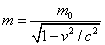to conserve y-direction momentum.

Note that this is an undetectably small effect at ordinary speeds, but as an object approaches the speed of light, the mass increases without limit!

Of course, we have taken a very special case here: a particular kind of collision.  The reader might well wonder if the same mass correction would work in other types of collision, for example a straight line collision in which a heavy object rear-ends a lighter object.  The algebra is straightforward, if tedious, and it is found that this mass correction factor does indeed ensure momentum conservation for any collision in all inertial frames.

### Mass Really Does Increase with Speed

Deciding that masses of objects must depend on speed like this seems a heavy price to pay to rescue conservation of momentum!  However, it is a prediction that is not difficult to check by experiment.  The first confirmation came in 1908, measuring the mass of fast electrons in a vacuum tube.  In fact, the electrons in an old-fashioned color TV tube are about half a percent heavier than electrons at rest, and this must be allowed for in calculating the magnetic fields used to guide them to the screen.

Much more dramatically, in modern particle accelerators very powerful electric fields are used to accelerate electrons, protons and other particles.  It is found in practice that these particles become heavier and heavier as the speed of light is approached, and hence need greater and greater forces for further acceleration.  Consequently, the speed of light is a natural absolute speed limit.  Particles are accelerated to speeds where their mass is thousands of times greater than their mass measured at rest, usually called the “rest mass”.

Warning: It should be mentioned that some people don’t like the statement that mass increases with speed, they feel that the word “mass” should be restricted to the rest mass of an object, which we’ve called m0.  This difference of definition has no physical content, however—it’s just a matter of taste.  We would write momentum as p = mv, they would write our m0 as m, and say the formula for momentum in their notation is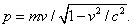Either way, a fast electron is that much harder to deflect from a straight line.

### Mass and Energy Conservation: Kinetic Energy and Mass for Very Fast Particles

As everyone has heard, in special relativity mass and energy are not separately conserved, in certain situations mass mcan be converted to energy mc2.  This equivalence is closely related to the mass increase with speed, as we shall see.  Suppose a constant force F accelerates a particle of rest mass min a straight line.  The work done by the force in accelerating the particle as it travels a distance d is Fd, and this work has given the particle kinetic energy.

As a warm up, recall the elementary derivation of the kinetic energy ½mv² of an ordinary non-relativistic (i.e. slow moving) object of mass m.  Suppose it starts from rest. Then after time t, it has traveled distance d = ½ at2, and v = at.  From Newton’s second law, F = ma, the work done by the force Fd = mad = ½ ma2t2 = ½ mv2

This won’t work if the mass is varying, because Newton’s Second Law isn’t always F = ma, for variable mass it’s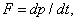force = rate of change of momentum, and if the mass changes the momentum changes, even at constant velocity.

An instructive extreme case is the kinetic energy of a particle traveling close to the speed of light, as particles do in accelerators.  In this regime, the change of speed with increasing momentum is negligible!  Instead,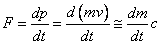where as usual c is the speed of light.  This is what happens in a particle accelerator for a charged particle in a constant electric field, with F = qE

Since the particle is moving at a speed very close to c, in time dt it will move cdt and the force will do work Fcdt.  The equation above can be rewritten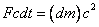So the energy dE expended by the accelerating force in the time dt yields an increase in mass, and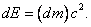Provided the speed is close to c, this can of course be integrated to an excellent approximation, to relate a finite particle mass change to the energy expended in accelerating it.

### Kinetic Energy and Mass for Slow Particles

Recall that to get momentum to be conserved in all inertial frames, we had to assume an increase of mass with speed by the factor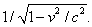This necessarily implies that even a slow-moving object has a tiny mass increase if it is put in motion.

How does this mass increase relate to the kinetic energy?  Consider a mass m0, moving at speed v, much less than the speed of light. Its kinetic energy E =½m0v², as discussed above. Its mass is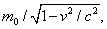which we can write as m0+dm, so dm is the tiny mass increase we know must occur.  It’s easy to calculate dm.

Forwe can make the approximations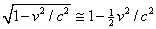and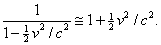So, for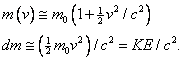Again, the mass increase dm is related to the kinetic energy KE by KE = (dm)c2.   Having looked at two simple cases, we’re ready to derive the general result, valid over the whole range of possible speeds.

### Kinetic Energy and Mass for Particles of Arbitrary Speed

We have shown in the two sections above that (in the two limiting cases) when a force does work to increase the kinetic energy of a particle it also causes the mass of the particle to increase by an amount equal to the increase in energy divided by c2. In fact this result is exactly true over the whole range of speed from zero to arbitrarily close to the speed of light, as we shall now demonstrate.

For a particle of rest mass m0 accelerating along a straight line (from rest) under a constant force F,Therefore, the work done when the particle moves a distance dx isusing v = dx/dt

Therefore the total work done from rest—the kinetic energy—is: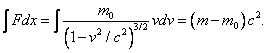(The integral is easily done by making the substitution y = v2/c2.)

So we see that in the general case the work done on the body, by definition its kinetic energy, is just equal to its mass increase multiplied by c2.

To understand why this isn’t noticed in everyday life, try an example, such as a jet airplane weighing 100 tons moving at 2,000mph.  100 tons is 100,000 kilograms, 2,000mph is about 1,000 meters per second.  That’s a kinetic energy ½mv² of ½.1011joules, but the corresponding mass change of the airplane down by the factor c² = 9.1016, giving an actual mass increase of about half a milligram, not too easy to detect!

### Notation: m and m0

As stated earlier, we use m0 to denote the “rest mass” of an object, and m to denote its relativistic mass,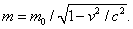In this notation, we follow French and Feynman.  Krane and Tipler, in contrast, use m for the rest mass.  Using m as we do gives neater formulas for momentum and energy, but is not without its dangers.  One must remember that m is nota constant, but a function of speed.  Also, one must remember that the relativistic kinetic energy is (m-m0)c2, and notequal to ½mv2, even with the relativistic mass!

Example: take v2/c2 = 0.99, find the kinetic energy, and compare it with ½mv2 (using the relativistic mass).

Category: Education | Views: 437 | Added by: farrel | | Rating: 0.0/0